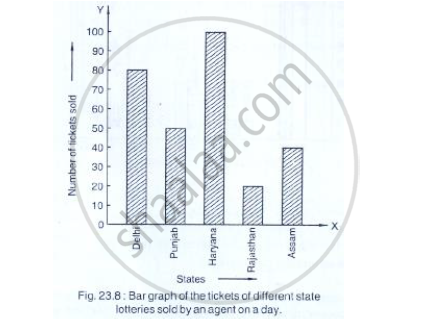# Read the Bar Graph Shown in Fig. 23.8 and Answer the Following Questions:What is the Information Given by the Bar Graph? (Ii) How Many Tickets of Assam State Lottery Were Sold by the Agent? - Mathematics

Read the bar graph shown in Fig. 23.8 and answer the following questions:(i) What is the information given by the bar graph?
(ii) How many tickets of Assam State Lottery were sold by the agent?
(iii) Of which state, were the maximum number of tickets sold?
(iv) State whether true or false.
The maximum number of tickets sold is three times the minimum number of tickets sold.
(v) Of which state were the minimum number of tickets sold?

#### Solution

(i) The given bar graph represents the number of the tickets of different state lotteries sold
by an agent on a day
(ii) Number of tickets of assam state lottery were sold by agent is 40
(iii) Haryana sold the maximum number of tickets
(iv) Minimum number of tickets sold = 20
Maximum number of tickets sold = 100
∴ 100=  5×20
So, the given statement is false
(v)The minimum numbers of tickets were sold is 20, in the state Rajasthan.

Concept: Graphical Representation of Data
Is there an error in this question or solution?

#### APPEARS IN

RD Sharma Mathematics for Class 9
Chapter 23 Graphical Representation of Statistical Data
Exercise 23.1 | Q 4 | Page 8

Share